Home > ACC7 > Chapter cc39 > Lesson cc39.2.3 > Problem9-91

9-91.
1.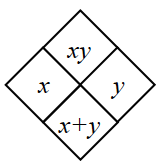Copy and complete each of the Diamond Problems below. The pattern used in the Diamond Problems is shown at right. Homework Help ✎

2.  a.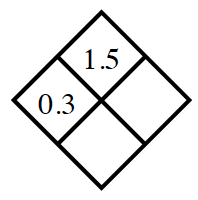b.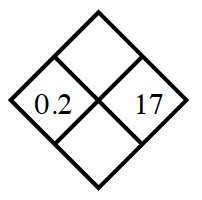c.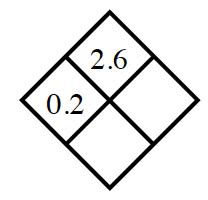d.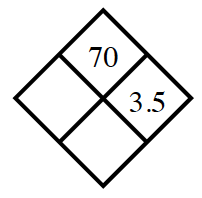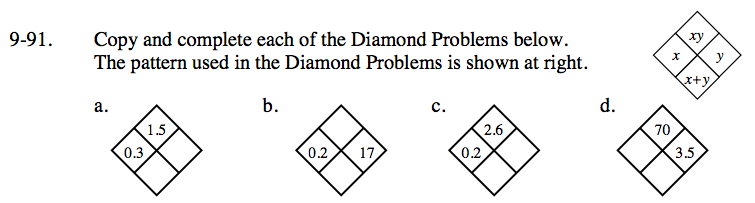What is 1.5 divided by 0.3?

Add x and y to fill the lower box.
Multiply them to fill the upper box.

What times 0.2 is equal to 2.6?

y = 13
Can you find the sum of 0.2 and 13?

This problem is very similar to part (c).